## Black-Scholes Model: First Steps

Today we take a look at the most popular options pricing model. The Black Scholes Model, also known as the Black-Scholes-Merton method, is a mathematical model for pricing option contracts. It works by estimating the variation in financial instruments. The technique relies on the assumption that prices follow a lognormal distribution. Based on this, it derives the value of an option.

It is more suitable for path-independent options, which the investors cannot exercise before their due date. This makes it different from the Binomial Option Pricing Model, which is a risk-neutral method for valuing path-dependent options (e.g., American options).

Analysts and investors consider Black Scholes to be one of the essential concepts in modern financial theory.

## Black Scholes Model

Introduced in 1973 in the Journal of Political Economy, by Fischer Black and Myron Scholes, and later built upon by Robert Merton, the model won the Nobel Prize in economics in 1997.

The model follows a differential equation, which we use to solve for options’ prices. We use the standard variation of the model for European style options. Black Scholes is not suitable for American style options, as it does not consider investors can exercise these options at any moment before their expiration date.

The method assumes the price of the underlying stock follows a lognormal distribution, as it cannot be negative. The distribution the model relies on is based on Brownian motion, a theory for predicting naturally occurring random phenomena.

We use the model to calculate an estimate for an option’s price, using the following information:

• The current price;
• The strike price;
• Time to expiration;
• Expected dividend yield;
• Expected interest rate; and
• Expected volatility.

Initially, the model doesn’t consider the effect of dividend payouts. Therefore it’s frequently adapted to do so by determining the ex-dividend date value of the underlying asset. The dividend yield has the effect of reducing the current stock price because the option holder forgoes the dividends.

### Assumptions

The Black Scholes model only works if we make certain assumptions.

• The model looks at European style options, which we can only execute at their expiration date;
• No dividend payments occur during the option life span (this was an assumption in the initial model, which analysts have since eliminated by adding the dividend yield in the model formula);
• The markets are completely efficient, meaning we can’t predict their movements;
• Buying and selling options involve no transaction costs;
• Both the volatility of the underlying asset and the risk-free rate remain constant until the expiration date, which is not correct, as in reality volatility shifts with supply and demand; and
• The returns of the underlying asset follow a normal distribution.

## Black Scholes Formula

The model formula is quite complex mathematically, which is not a particular issue, as we can employ specialized tools to calculate it for us. But it’s still essential to understand how it works.

To calculate the price of a call option, under the Black Scholes model, we can use the following equation:

Where:

• S0 is the stock price;
• e is the exponential number;
• q is the dividend yield percentage;
• T is the term (one year will be T=1, while six months will be T=0.5);
• N(d1) is the delta of the call option, meaning the change in the call price over the shift in the stock price;
• K is the strike price;
• r is the risk-free rate; and
• N(d2) is the probability that the future stock price will be higher than the strike price, the likelihood we will exercise the option.

One side is the stock price multiplied by the cumulative standard normal distribution of d1, and the other – the strike price, discounted to time T and then multiplied by the cumulative standard normal distribution of d2.

Where we can calculate d1 and d2 with the following formulae: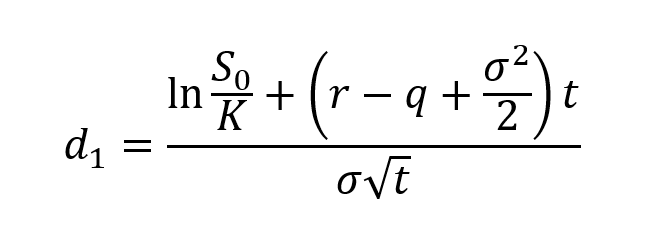Where σ is the volatility of the returns of the underlying asset.

We can apply the same logic for a put option: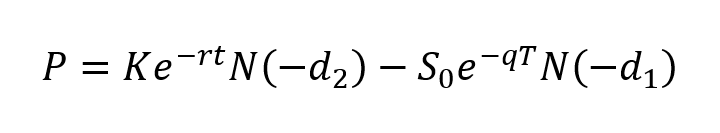## Example Black Scholes Calculation

To better illustrate the concept behind the Black Scholes Model, we will take a look at the following model. We start with the model inputs. As discussed above, we need the following six variables to calculate the call and put option values.Next, we will calculate the options’ parameters. Starting with d1, we get 0.39 from our model assumptions.

To calculate it, we simply transfer the formula for d1 to Excel.

Following the same approach, we calculate d2, N(d1) and N(d2).

With those, we can calculate the Call Option Price and the Put Option Price.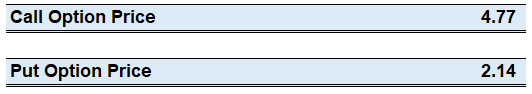We can employ the Call-Put Parity rule to check whether our formulas and calculations are correct.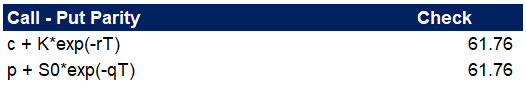Now that we have our model to calculate the Call and Put options prices, we can go a step further and look at how the different input parameters affect the results of our model. The Data Table functionality in Excel is a great tool to prepare some sensitivity analysis tables for our variables. You can learn how to use Data Tables in our Sensitivity Analysis article.

Let’s look at the Stock Price if it changes from 10 to 100 and how it affects the Call and Put values. We notice that the Call Option has no value up until the stock price hits 40. We see the reverse for the Put Option, where its value hits zero once the stock price goes above 85.If we prepare the same sensitivity table for the Strike Price, we can observe the reverse relationship. As the Strike Price increases, the Call Value goes down, and the Put Value goes up.Let’s also take a look at the risk-free rate. As it increases, this also increases the required rate of returns. Subsequently, this decreases the value of stocks. We notice a direct relationship, as the risk-free rate increases, the Call Option value increases as well, while the Put Option value decreases its worth.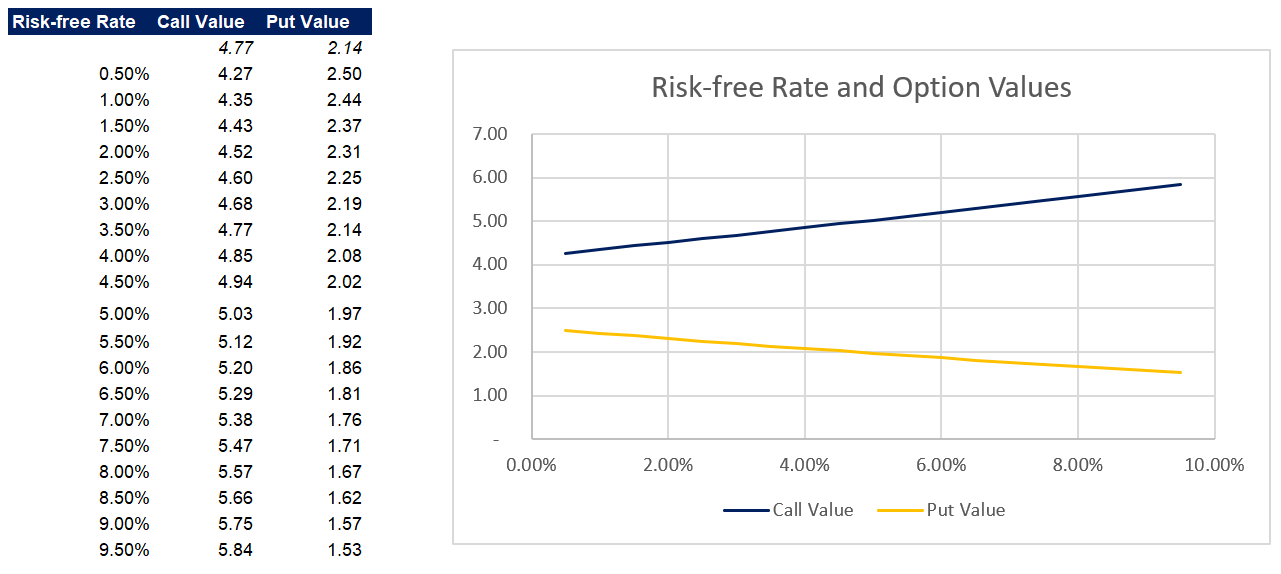Following the same approach, you can go ahead and look at the rest of the parameters and how they affect the Option Values. You can download the Excel model at the end of the article and go over the sensitivity analysis in there.

## Conclusion

It is important to remember that the Black Scholes Merton model is a theoretical concept. We use it to try and estimate the behavior of the market. However, when we use the results in our decision-making process, we have to understand the underlying assumptions in the model and how it deviates from reality.

The formula helped options trading to become more popular, as it makes it look less like gambling. Nowadays, various modifications of Black Scholes are widely popular as fundamental strategies for risk control, associated with volatility.

You can show your support by sharing the article with colleagues and friends. Also, don’t forget to download the example Excel model below.

## Get a FREE Excel Benchmark Analysis Template## FCCA, FMVA

Hi! I am a finance professional with 10+ years of experience in audit, controlling, reporting, financial analysis and modeling. I am excited to delve deep into specifics of various industries, where I can identify the best solutions for clients I work with.

In my spare time, I am into skiing, hiking and running. I am also active on Instagram and YouTube, where I try different ways to express my creative side.

The information and views set out in this publication are those of the author(s) and do not necessarily reflect the official opinion of Magnimetrics. Neither Magnimetrics nor any person acting on their behalf may be held responsible for the use which may be made of the information contained herein. The information in this article is for educational purposes only and should not be treated as professional advice. Magnimetrics and the author of this publication accept no responsibility for any damages or losses sustained in the result of using the information presented in the publication.